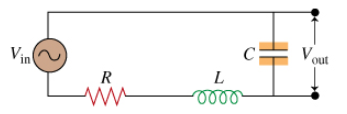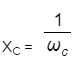# Problem: What is the ratio Vout/Vin? Express your answer in terms of either R, ω, L, and C or R, XL =ωL, and

###### FREE Expert Solution

Current in circuit, i = Vin/Z = Vin/sqrt[R2 + (XL - XC)2]

Output voltage, Vout = i sqrt(R2 + XL2) =[ Vin/sqrt{R2 + (XL - XC)2]sqrt(R2 + XL2)

100% (315 ratings)###### Problem DetailsWhat is the ratio Vout/Vin? Express your answer in terms of either R, ω, L, and C or R, XLL, and